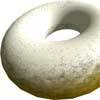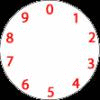#### You may also like### Doughnut

How can you cut a doughnut into 8 equal pieces with only three cuts of a knife?### Rectangle Tangle

The large rectangle is divided into a series of smaller quadrilaterals and triangles. Can you untangle what fractional part is represented by each of the shapes?### Dividing a Cake

Annie cut this numbered cake into 3 pieces with 3 cuts so that the numbers on each piece added to the same total. Where were the cuts and what fraction of the whole cake was each piece?

# Light Blue - Dark Blue

##### Age 7 to 11 Challenge Level:

Francesca investigated this problem. She imagined that each time the big square was split up into little blocks that looked like the light blue ones. Then she counted how many light blue ones there were, and how many overall. This is what she got :

1, $\frac{2}{3}$, $\frac{4}{9}$, $\frac{8}{27}$, $\frac{16}{81}$, $\ldots$

She noticed that the number on top got multiplied by 2 each time, and the number on the bottom got multiplied by 3 each time.
Some of our more advanced readers might know that we could write this as

$\frac{2^n}{3^n}$.

Francesca also noticed that the amount of light blue got smaller and smaller each time. She thinks that if we could do this forever, in the end the whole square would be dark blue.